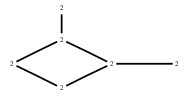# The Markov Bases Database

## G98_bin

The binary graphical model/graph model of G98.

It is a hierarchical model of 6 variables. The dimension of the model is 12.The cardinality of the statespace is 64.

### Properties of the Markov basis

 Markov degree 4 1184

 degree # of gen. 2 4 536 648

The model has the following properties:

• All variables are binary.
• It is a graph model and a graphical model.
• The semigroup is normal.

The following properties of the model are unknown:

• The minimal Markov basis may be unique.### FILES

 Markov basis: sufficient statistics matrix: G98_bin.mar (223.16 kb) G98_bin.mat (3.03 kb) G98_bin.mod (53 b) G98_bin.tar.gz (11.65 kb)

Wrong or missing information? Write us an email.

page last update on November 15 2020. ->Contact